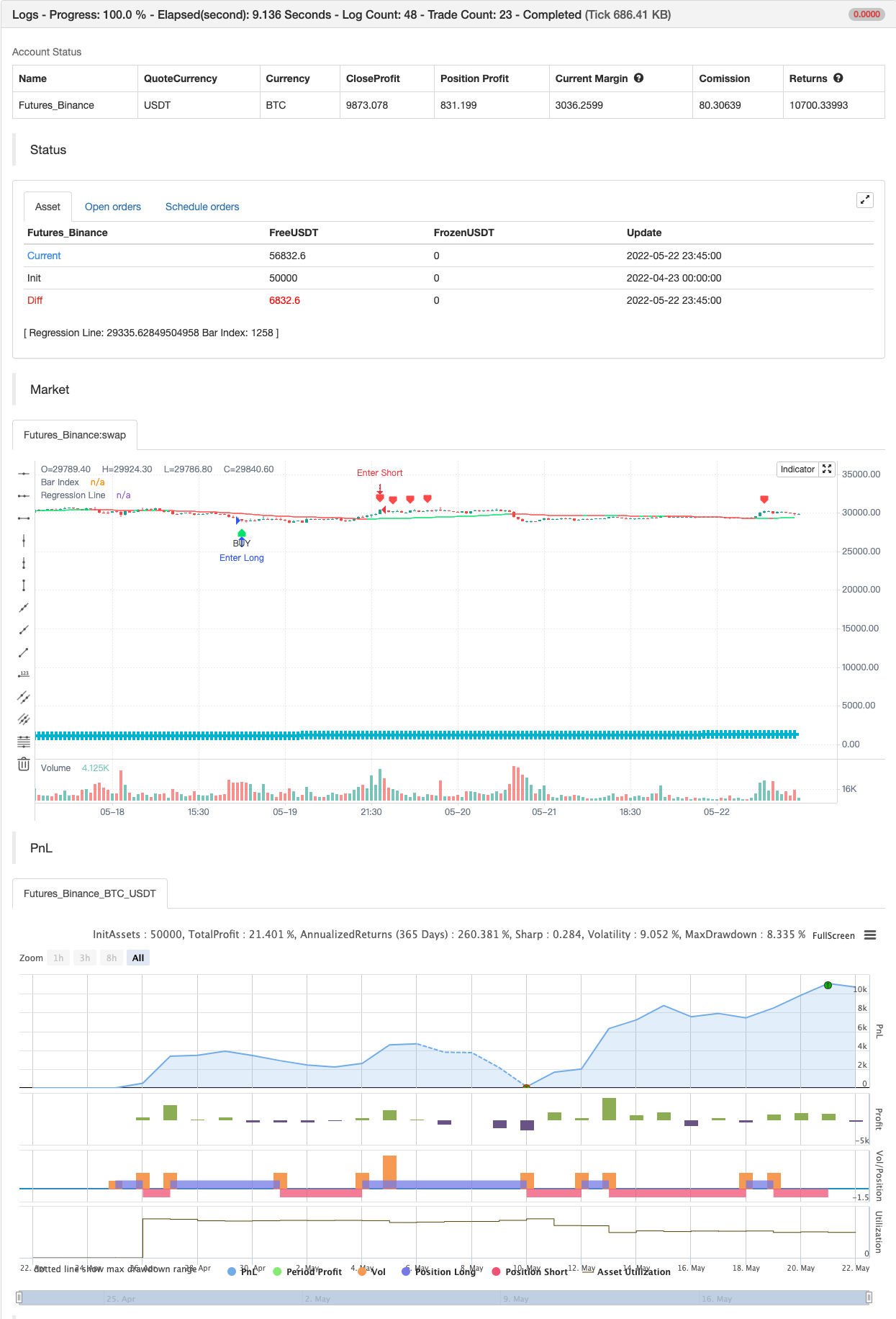# Linear Regression ++

Author: 张超, Date: 2022-05-24 14:17:42
Tags: DEV

ue to public demand

Linear Regression Formula Scraped Calculation With Alerts

Here is the Linear Regression Script For traders Who love rich features

Features ++ Multi time frame -> Source Regression from a different Chart ++ Customized Colors -> This includes the pine lines ++ Smoothing -> Allow Filtered Regression; Note: Using 1 Defaults to the original line. The default is 1 ++ Alerts On Channel/Range Crossing

Usage ++ Use this for BreakOuts and Reversals ++ This Script is not to be used Independently

Risks Please note, this script is the likes of Bollinger bands and poses a risk of falling in a trend range. Signals may Keep running on the same direction while the market is reversing.

Requests If you have any feature requests, comment below or dm me. I will answer when i can. Feel free to utilize this on your chart and share your ideas

For developers who want to use this on their chart, Please use this script The original formula for calculation is posted there

❤❤❤ I hope you love this. From my heart! ❤❤❤

backtest```/*backtest
start: 2022-04-23 00:00:00
end: 2022-05-22 23:59:00
period: 45m
basePeriod: 5m
exchanges: [{"eid":"Futures_Binance","currency":"BTC_USDT"}]
*/

// This source code is subject to the terms of the Mozilla Public License 2.0 at https://mozilla.org/MPL/2.0/
// User Version

//@version=4
study("Linear Regression ++ [Lucem Anb]", "Lin Reg ++ [Lucem Anb]", overlay=true)

source      = input(close)
length      = input(100, minval=1)
offset      = input(0, minval=0)
dev         = input(2.0, "Deviation")
smoothing   = input(1, minval=1)
mtf_val     = input("", "Resolution", input.resolution)
line_thick  = input(4, "S&R Thickness", minval=1, maxval=4)
signals     = input("Recent", "Signals Display", options=["Recent", "All"])
p           = input("Lime", "Up Color", options=["Red", "Lime", "Orange", "Teal", "Yellow", "White", "Black"])
q           = input("Red", "Down Color", options=["Red", "Lime", "Orange", "Teal", "Yellow", "White", "Black"])
goto        = input(0, "End At Bar Index")

cc(x) => x=="Red"?color.red:x=="Lime"?color.lime:x=="Orange"?color.orange:x=="Teal"?
color.teal:x=="Yellow"?color.yellow:x=="Black"?color.black:color.white
data(x) => sma(security(syminfo.tickerid, mtf_val!="" ? mtf_val : timeframe.period, x), smoothing)

linreg = data(linreg(source, length, offset))
linreg_p = data(linreg(source, length, offset+1))
plot(linreg, "Regression Line", cc(linreg>linreg?p:q), editable=false)

x = bar_index
slope = linreg - linreg_p
intercept = linreg - x*slope
deviationSum = 0.0
for i=0 to length-1
deviationSum:= deviationSum + pow(source[i]-(slope*(x-i)+intercept), 2)
deviation = sqrt(deviationSum/(length))
x1 = x-length
x2 = x
y1 = slope*(x-length)+intercept
y2 = linreg

updating = goto <= 0 or x < goto

dm_current = -deviation*dev + y2
dp_current = deviation*dev + y2
sell = crossover(close, dp_current)
alertcondition(sell, "Sell Lin Reg", "Crossing On the Higher Regression Channel")